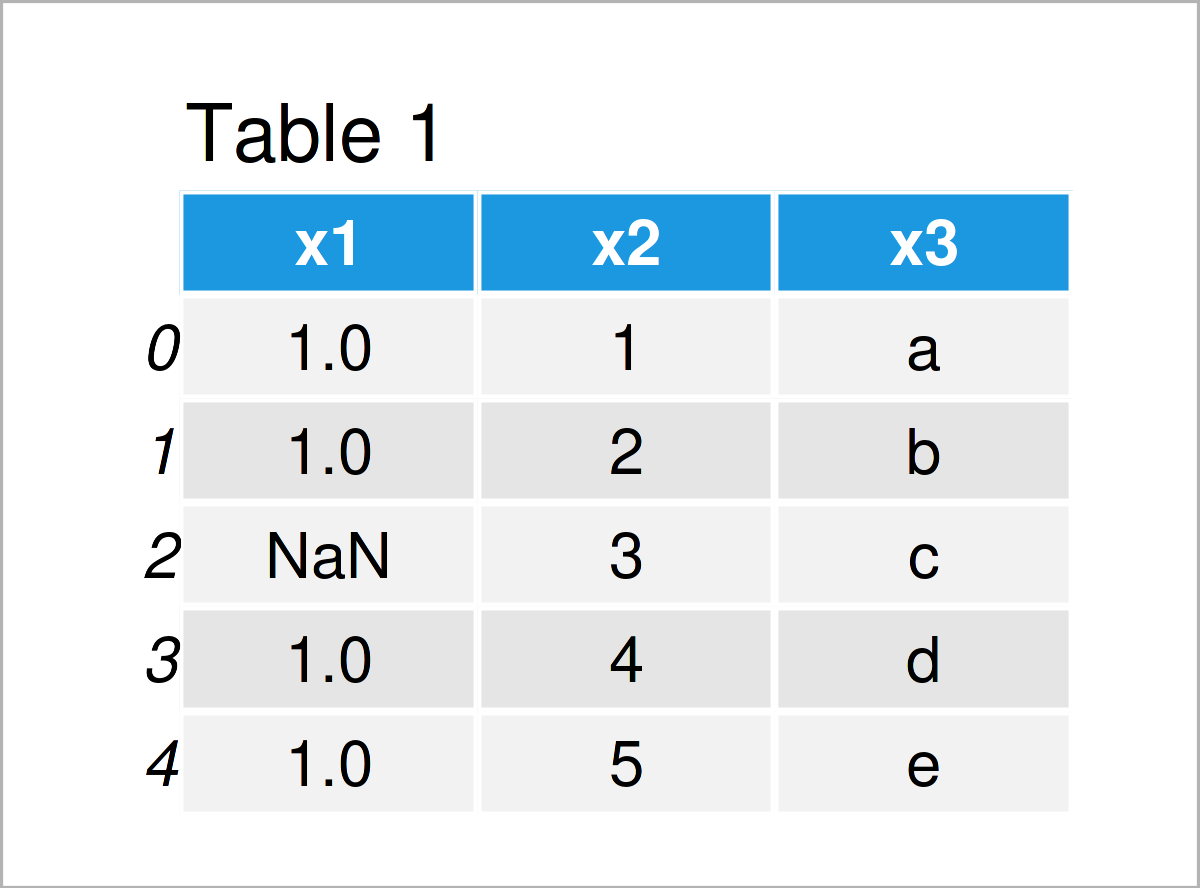# Check If Any Value is NaN in pandas DataFrame in Python (Example)

In this Python tutorial you’ll learn how to test for NaN values in a pandas DataFrame.

The content of the tutorial is structured as follows:

## Exemplifying Data & Add-On Libraries

If we want to use the functions and commands of the pandas library, we first need to import pandas:

`import pandas as pd # Import pandas`

We use the following data as basement for this Python programming tutorial:

```data = pd.DataFrame({'x1':[1, 1, float('NaN'), 1, 1], # Create example DataFrame 'x2':range(1, 6), 'x3':['a', 'b', 'c', 'd', 'e']}) print(data) # Print example DataFrame```Table 1 shows that our example data is composed of five rows and the three variables “x1”, “x2”, and “x3”.

As you may already have seen in Table 1, our pandas DataFrame contains one NaN value (i.e. a missing value; Not a Number) in the first column.

However, let’s check that by using some Python code!

## Example: Test Whether pandas DataFrame Contains NaN Values Using isnull() & any() Functions

This example illustrates how to check if any data cell in a pandas DataFrame is NaN.

For this task, we can apply the isnull and any functions in combination with the values attribute as you can see below:

```print(data.isnull().values.any()) # Apply isnull & any # True```

After running the previous Python syntax, the logical value True is returned. In other words: Our example data contains at least one NaN value.

## Video & Further Resources

Do you need more info on the Python programming codes of this tutorial? Then you may watch the following video on the Data School YouTube channel. In the video, the speaker demonstrates how to handle missing values in Python.

Please accept YouTube cookies to play this video. By accepting you will be accessing content from YouTube, a service provided by an external third party.If you accept this notice, your choice will be saved and the page will refresh.

Besides that, you might want to read the related tutorials on this homepage:

You have learned in this article how to check for NaN values in a pandas DataFrame in Python. In case you have additional questions, don’t hesitate to let me know in the comments section.

Subscribe to the Statistics Globe Newsletter